Refer to our Texas Go Math Grade 2 Answer Key Pdf to score good marks in the exams. Test yourself by practicing the problems from Texas Go Math Grade 2 Module 7 Assessment Answer Key.

Concepts and Skills

Write the sum. (TEKS 2.4.B)

Question 1.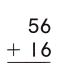Explanation:
56 + 16 = 72
The sum of 56 and 16 is 72

Question 2.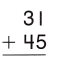Explanation:
31 + 45 = 76
The sum of 31 and 45 is 76

Question 3.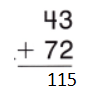Explanation:
43 + 72 = 115
The sum of 43and 72 is 115

Write a number sentence for the problem. Use a ☐ for the missing number. Then solve. (TEKS 2.4.C)

Question 4.
Jake had some stamps. Then he bought 20 more stamps. Now he has 56 stamps. How many stamps did Jake have to start?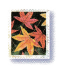___________ stamps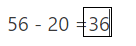Explanation:
Then he bought 20 more stamps.
Now he has 56 stamps.
Missing number is 36

Question 5.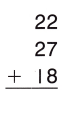Explanation:
The sum of 22, 27 and 18 is 67

Question 6.Explanation:
The sum of three numbers is 35,24 and 58 is 117

Question 7.Explanation:
The sum of 60 + 15 + 3 + 21 = 99

Question 8.Explanation:
The sum of 58 + 12 + 24 + 64 = 158

Fill in the bubble for the correct answer choice.

Question 9.
Miles saw some toy cars at the store. 16 of the cars are red and 18 of the cars are green. Which number sentence could be used to find how many cars he saw? (TEKS 2.4.C)
(A) 16 + ☐ = 18
(B) 16 + 18 = ☐
(C) ☐ + 16 = 18
Explanation:
16 + 18 = ☐ is used to find the equation
16 + 18 = 34

Question 10.
Avery had 60 crayons. She bought 2 more crayons. Then Edward gave her 33 crayons. How many crayons does Avery have now? (TEKS 2.4.B)(A) 108
(B) 75
(C) 95
Explanation:
She bought 2 more crayons.
Then Edward gave her 33 crayons.
95 crayons that Avery have now
60 + 2 + 33 = 95

Question 11.
Gavin has 54 coins. His sister gives him 36 coins. How many coins does he have now? (TEKS 2.4.B)
(A) 80
(B) 18
(C) 90
Explanation:
Gavin has 54 coins.
His sister gives him 36 coins.
54 + 36 = 90
90 coins that he have now

Question 12.
(A) 119
(B) 115
(C) 129
Explanation:

Question 13.
Parker collected 21 shells. Katie collected 34 shells. Ella collected 15 shells. Marco collected 26 shells. How many shells did the four children collect? (TEKS 2.4.B)(A) 70
(B) 96
(C) 86